main page   list of papers   library

c Copyright - Karim A. Khaidarov, January 30, 2004.

AETHEREAL ATOM

Dedicated to the bright memory of my daughter Anastasia

Discovery is seeing what everybody else has seen,
and thinking what nobody else has thought.

A. Szent-Gyorgyi

Taking as a fact  the presence of the ether in the Universe, united quasi-isotropic, practically incondensible and ideal elastic medium, being the main basic matter, the carrier of the whole energy, all processes, occurring in the Universe, and taking for the base of beliefs about it working model [2-6], presenting it in the manner of twocomponent medium - corpuscular and phase, we will consider some questions of atoms forming in the ether.

The Dynamic Density of the Ether

"As is well known", atom is practically empty, that is to say practically whole of its mass and energy are concentrated in the nucleus. The size of nucleus in 100000 times less than atom itself. What is a matter which fills this emptiness? And so that the last bears whole mechanical load and simultaneously can be an ideal conductor of the light?

Let's consider dependency of refraction factor in transparent materials, shown on figure 1.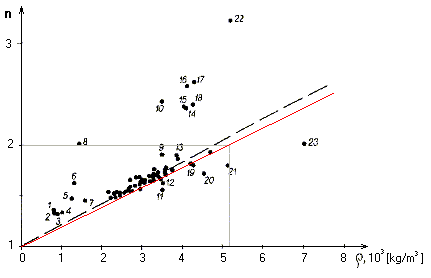Fig. 1. The dependency of refraction factor from density of material, built by F.F. Gorbatzevich in  on data from [8, 9].
(Red line is a share of refraction, explained by density of all electrons of substance. 1 - ice, 2 - acetone, 3 - alcohol, 4 - water, 5 - glycerine, 6 - sulfurcarbon, 7 - 4-chlorid carbon, 8 - sulphur, 9 - titanit, 10 - diamond, 11 - grotit, 12 - topaz.)

F. F. Gorbatzevich  gives following empirical dependency of mass density of substance ρs and factor of refraction n for transparent materials

 n = 1 + 0.2 ρs (1)

This dependency is reflected in dotted line on figure 1. However if we take that according to proposed by author in  models of the ether, the last has dynamic density, uniquely connected with the speed of light in medium, and consequently, with factor of refraction, that in first approximation it is possible to explain data shown on figure 1 by following formula (red line on figure 1)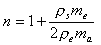(2)

where ρs – is a density of transparent material;
ρe – is dynamical density of the ether, discovered in ;
me – is a mass of electron;
ma – is the atom unit of mass.

From (2) with the whole conspicuity follows that practically whole volume of material forms by electrons and growth of dynamic density of ether for light wave corresponds to growth of electrostatic density (elecrostrictional, potential energy) of electrons, expressing in growing of a dielectrical permeability of ether in material. Let's try to understand, what it is.

Domain Model of the Ether

In works [1-6] the working model of ether reducing to the following thesises is developed.

The ether consists of amers, spherical, elastic, practically incondensable protoelements having size of 1.616 ·10-35 [m], and having characteristics of ideal giroscope weel with internal energy 1.956 ·109 [J].

The main part of amers are still and collected in ethereal domains, having usual ether temperature 2.7 oK with size, commensurable to size of classical electron. Under this temperature there are 2.708 +1063 amers in each domain. The domains size defines from ether polarization i.e. velocity of light in the ether. When the size of domain increase, light velocity falls, since linear dielectric and in some cases magnetic permeability of ether increase. When the temperature of ether increase, the size of domains decreases, and velocity of light increases. The ethereal domains have high surface tension force.

Free amers, presenting by itself the phase ether and placing between ethereal domains, move with local velocity of light, definied by temperature of the ether. The ensemble of amers of phase ether, moving with statistical average velocity, corresponding to local second space velocity, reflecting gravitational potential, provides functioning of the mechanism of sources - discharges in three-dimensional space.

Real gravitational potential creates by the variations of ether pressure, absolute value of which is 2.126+1081 [kg/ms2], and presents itself as usual hydrostatic pressure, acting with longitudinal (sonic) velocity of ether ("fast gravitation" in author's term).

Interdomain borders in the ether are monoamereal i.e. having the thickness of one amer or less, up to density of substance, comparable with nuclear density. The phase ether is a measure of gravitational mass of substance and is accumulated in substance, in nucleons in proportion 5.01+1070 [vac/kg] i.e. amers of phase airwaves per kg. In that time, as domains of empty ether present itself original pseudo-liquid, nucleon presents itself as boiling domain of ether, containing main share of phase ether and, accordingly to that, gravitational mass.

According to developing model of the ether electrons present itself electrified ethereal domains of low temperature, residing in pseudo-liquid condition and having borders with high power of surface pull, which is property of all ether domains under its usual low temperature 2.7 oK.

Neutrino are interpretted as ethereal phonons , generated by ethereal domains and spreading both with transverse velocity of the ether - velocity of light, and with longitudal velocity - the velocity of fast gravitation.

The Model of Electron in Domain Ether

As there was shown in , electron presents itself charged ethereal domain, inwardly which the standing electromagnetic wave circulates, reflecting from walls of domain. At moment of forming the electron, as there was shown in , has the classical radius - 2.82 +10-15 [m], commensurable to size of domain in empty ether. The electric potential of surface of electron at this moment is 511 kV. However such parameters are not stable, and after few time electrostatic power sprains domained electron in original very fine lens, which size are defined by power of surface pull of domain. On equipotential and, consequently, superconducting perimeter of this lens takes seats the electric charge of electron, spraining this domain (fig. 2).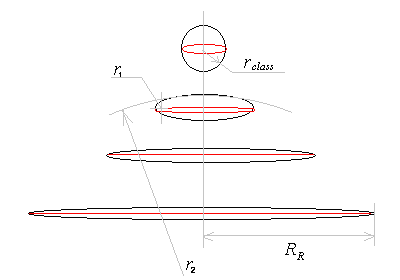Fig. 2. The change the form of electron after its origin.

Considering surface pull σ of ethereal domain and counting balance of this power with power of electrostatic sprain of charged domain, creating a pressure Δp by the law of Laplace [P. S. Laplace, 1806]

 Δp = σ (1/r1 + 1/r2), (3)

in absence of external electric field and motion of electron comparatively surrounding phase ether, it is possible to define the radius of electron by following formula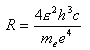(4)

where ε – is ether dielectric permeability;
h – is a Planck constant;
c – is a light velocity;
me – is a mass of electron;
e – is a charge of electron.

The value (4) equals 1/2 Rydberg constant in empty ether. Inwardly such disk - domain the standing electromagnetic wave circulates, It has, as shown in , wavelength, equal four radii of disk so crest of waves account to the centre of this disk - resonator, but the nodes of waves placed on its periphery. Since dynamic density of the ether inwardly such domain varies inversely with square of radius of disk, that velocity of electromagnetic wave propagation in electron's body is such, that quarter of wave packs exactly in this radius always. Thereby condition of resonance is kept always. Since density inside such domain is always higher than dynamic density of surrounding ether, but angle of incidence of wave practically is a zero, that the phenomena of full internal reflection exists.

Depending on external electrostatic field, being equipotential, the rim of disk - electron always turns around on normals to vector of field. The turn can be as to one, so and to another side, that is to say "spin" of electron can be +1/2 or -1/2. Besides that, radius of electron strictly depends on tension of electrostatic field, since the joining power in electron corresponds to tension of this field. This effect appears therefore that standing electromagnetic wave is an centro-symmetrial electric dipole, which tries to turn round on vector of electrostatic field. In absence of external hanhold and in connection to variable nature of electromagnetic field it brings only the origin of centripetal power, changing the radius of disk as 

 R = τ/2εE [m], (5)

where ε – is an ether dielectric permeability [F/m];
τ – is a linear charge density [C/m];
c – is a light velocity [m/s];
me – is a mass of electron [kg];
e – ia a charge of electron [C]
E – is intensity of electrostatic field [V/m].

Equation (5) exactly fits to experimental data of measurement of section of electrons capture midair .

Thereby offered model of electron complies with models of electron as whorl of current, developped in works of Kenneth Snelson , Johann Kern  and Dmitri Kozhevnikov  and developped by them models of atom.

Light Wave in Transparent Substance

It is known that atoms in solids and liquids are located tightly to one another. If electrons, by density of which absorbances of material is defined, move on orbits, as that provided by Bohr's atom model, that even under elastic interaction with electrons already at passing several atomic layers of material a light gains dispersional nature. Really, in transparent materials we see absolutely other picture. The light does not lose their own phase features due passing more than 1010 atomic layers of material. Consequently, electrons not only do not move on orbits, but exceedingly still, as that can be under the temperature close to absolute zero. There is exactly so. The temperature of electrons in transparent material does not exceed a temperature of ether, 2.7oK. Thereby usual phenomena of materials transparency is a disclaimer of existing atom model.

The Model of Aethereal Atom

In this connection we try to create the own atom model, resting only in obvious properties of electron in model proposed above. At first we define that the main acting forces in volume of atom, that is to say outside of the measly size nucleus, are:

• the interaction of central electrostatic force of nucleus, proportional to amount of protrons, with electrostatic force of electrons;
• interferentional interaction of electromagnetic field of nucleus on current loops of electrons;
• magnetic force of interaction of electrons current loops (its "spins") between itself.

For profound consideration of process of electrostatic interaction of nucleus and electrons we will consider the quantitative feature of electrostatic force. Central force of nucleus is expressed in its electric field intensity

 E = Ae/4πεr2 [V/m], (6)

where A – is amount of protons in nucleus;
e - is electron charge [C];
ε – is a dielectric permeability of the ether [F/m];
r – is a distance from nucleus [m].

Any electron in central field (atom inside, in absence of electric field of other atoms), being equipotential, is situated greatly sprawlling up to semisphere or until contact with other electron. Its possibility to sprawl up to Rydberg radius is not consider, since this value in 1000 times greater size of atom. Thereby simplest atom of Hydrogen has the form, shown on figure 3a, and atom of Helium - 3b.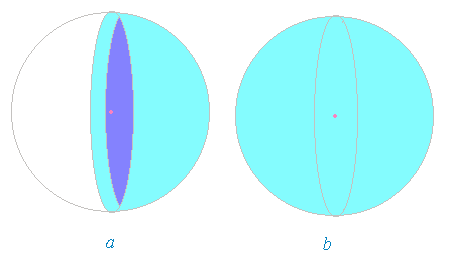Fig.3. Hydrogen and Helium atoms model.

Really, edges of electron - semisphere in atom of hydrogen mildly elated, since here reveals itself the marginal effect. The Helium atom is so tightly locked by shell of two electrons, that it is exceedingly inert material. Unlike Hydrogen it has no electric dipole properties. Easy notice, that in atom of Helium edges of electrons can be press in that case only if electrons have directions of electric current in its rims coincides, that is to say they have the opposite spins.

The electric interaction of electrons edges and magnetic interaction of its surfaces is one more mechanism, acting in atom.

In works of Kenneth Snelson , J. Kern , D. Kozhevnikov  and other researchers the main stable configurations of electron atom shells with model of electron of "current loop - magnet" type is elaborated. The main stable desksides are 2, 8, 12, 18, 32 electrons in shell, providing symmetry and maximum closing electric and magnetic forces.

Resonance Electromagnetic Interference of Electrons and Nucleus

As we know that protron has a charge moving on its volume, it is easy to find that this circumstance creates electromagnetic field in space around protron. Since frequency of this field too high, its spreading outside of atom (10-9 m) is measily and does not carry away any energy. However near protron (atom nucleus) its existing essential tension composes interferential picture.

Minimums of tension of this interference for atom of Hydrogen will correspond to step, equal to Bohr's radius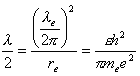(7)

where λe – is electrons characteristic wave length;
re – is a classic electron radius;
ε - is an ether dielectric permeability;
h – is Planck's constant;
me – is electron mass;
e – is electron charge.

The electric current loops of electrons are displaced by this field into these niches, corresponding to radiuses of electronic shells of atom. Thereby "quantum" conditions of electrons in atom appear. On figure 4 simplified dependency of complex force field, acting on electrons in atom, is shown.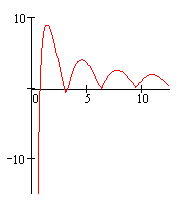Fig. 4. Simplified univariate scheme of distribution the complex force field of atom

Mendeleev's Table

Using equation for central electrostatic field (6), influence of interference (7) and aproximate calculation of electrostatic and magnetic interaction of electrons, author built a table of electronic shells for chemical elements from number 1 to 94.

This table some differs from usual. However, considering invalidity of orbital theory of Bohr and Schrödinger's presentation of electron, as a wave of probability, it is difficult to say what table is more closer to truth.

Amust to note, that by using this table, it is possible to get the radii of atoms, which are defined by amount of shells and its energy condition. The radius of valence atom in material is to one shell less or more, depending on returning or taking electrons.

Simplified formula for radius of atom is following

Ra = RBn3/Z

where Ra – is atom radius;
RB = λ/2 – is semiwave of elementary resonance (7), Bohr's radius;
n – is amount of electronic shells (depends of current valency);
Z – is amount of protons in nucleus.

Thereby for density of transparent material it is possible to give greatly more exact formula, than (1) or (2)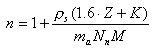(8)

where ρs is density of transparent substance;
ma = 1.66 ·10-27 [kg/nuc] – is an atom unit of mass.
Z – is amount of protons in molecule;
N = 3/R3 = 1.6 ·1030 [nuc/ m3] – is amount of nucleons in 1 m3 counting Bohr's radius; M - is a molecular weight;
K – is the factor of reduction or increasing the volume of molecule to account of loss or aquisition of atom valence shell.

The factor K equals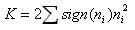Sum is doing on all i-atoms of molecule. Values n, found by author for elements of Mendeleev's table, are provided in table.

Validation of Theoretical Model on Transparent Materials

Using formula (8) it is possible to find the proper value of absorbance (the factor of refraction) of substances. Conversely, if we know the factor of refraction and chemical formula, it is possible to calculate the proper value of mass density of material.

The author analysed more than hundred of different materials: organic and inorganic. Computable on formula (8) factor of refraction is compared with measurable. The results of comparison show that dispersion of data less than 0.0003, and correlation factor higher than 0.995. The source dependency of mass density of material from factor of refraction is shown on figure 5, and dependency of theoretical factor of refraction from measured - on figure 6.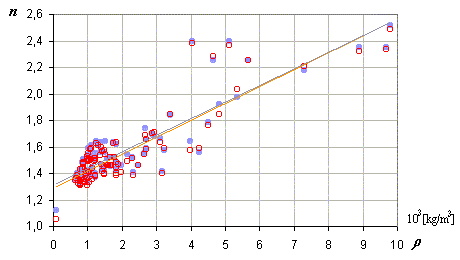Fig. 5. The dependency of factor of refraction from density of material
(blue points are measured values, red circles - calculated values)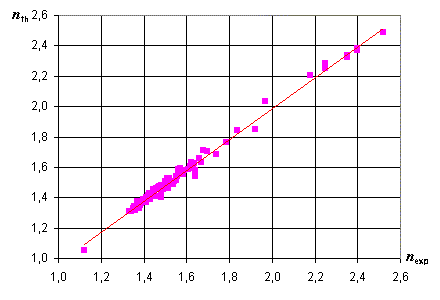Fig. 6. The dependency of theoretical factor of refraction from measurable.

Validation of Theoretical Model on Electronograms

Interpretation of electronograms according to proposed atom model is reduced to that is no diffraction of "slow" electrons, but there are simply its reflection from surface layer of material or refraction in fine layer.

Let's consider typical electronograms of metals: copper, silver and gold (fig. 7).

There is obviously seen that its are an image of still electronic shells. Moreover we can define the thickness of electronic shells and its placement upon atom radius. Naturally, that distances between shells are garbled by the voltage (the energy) of bombarding electrons. However proportions between intershells gaps and thicknesses of shells are saved.

Besides, it is seen that thicknesses of shells (amounts of electrons) more correspond to Bourabai model of atom, then Bohr's model ;-).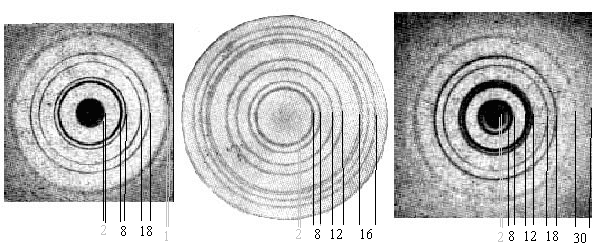Fig. 7. The Electronograms of metalls Cu, Ag, Au.
(Electron distribution Cu 2:8:18:1, Ag 2:8:12:16:8:1, Au 2:8:12:18:30:8:1)

Given electronograms are not a diffraction, but only a picture of reflection of bombarding electrons from electronic shells, which are still in general case. According to proposed model the visible thickness of ethereal domains - electrons in atom is a constant. So, on type of reflections (not a diffraction) it is possible to evaluate the thickness and location of each electronic shell. On figure 7 there is distinctly seen the stratification of fourth shell of silver atom, under influence of bombing, on 3 sub-shells: 2-6-8. The most powerful stratification is seen in external valence shells and uncompleted, which have a minimum stability (the author names such shells "active"). This is well seen on example of classical aluminum electronogram, when energy of bombarding electrons is different (fig. 8).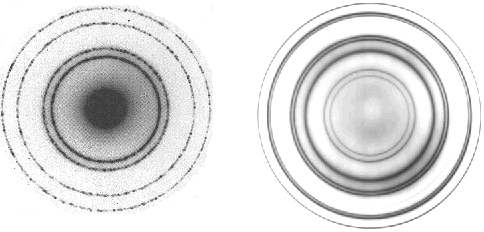Fig. 8. Aluminum electronograms under different energy of bombing electrons.

Variation of Light Velocity in Atom

Unfilling of some shells in atom until stable set causes the mobility of electrons. As a result this interferential niches of electromagnetic field of nucleus, in which these electrons are locate, have low ether dynamic density (the increased ether temperature).

Two these factors bring about everyday observed, but wrong interpretted phenomena - light mirror reflection by metallic surfaces.

The reason of mistake is the same dogmatic faith in mythic constancy of light velocity, even in that cases, when this disagrees to stated centuries ago simple and clear conclusion. It is known, that for any media and waves an attitude of velocity back pro rata wave density (and optical too).

sin (i)/sin(r) = c1/c2 = n2/n1 = n21

where i – is an angle of incidence; r – is an angle of refraction; c1- is a wave velocity in incidence medium; c2 – is a wave velocity in refraction medium; n2 – is an index of refraction of second medium; n1 – is an index of refraction of first medium; n21 – is a relative index of refraction.

Thence, it does not follow any restriction on velocity, and if we get in experiment that factor of refraction of medium lower than 1, that is logistical to confirm that velocity in this medium is higher than in that, in which we consider n = 1. In this case equation n = 1 is conditional itself, and refers only to present state of medium (the ether). As there was shown by author in  under other thermodynamic conditions an ether has other density, other temperature and other velocity of waves propagation. Naturally, in the case of unfilled, active electronic shells it is necessary to take into account a second order factor - speedup of electrons irradiated by light, that is to say absorption, dispersion and connected with it imaginary component of refraction factor. Consolidating all to this factor of the second order it is possible to come to paradoxes, which we see in physics of 20-th century.

Thinking so, author has analysed the different materials on velocity of light in its external electronic shells. The results of that are provided in the following table.

Table 2. The velocities of the light propagation in surface layer of metals.

 Metall Simbol n d [1000 kg/m3] c [1000 km/s] Sodium Na 0,050 0,966 6000 Caesium Cs 0,095 1,900 3158 Silver Ag 0,120 10,500 2500 Copper Cu 0,180 8,900 1667 Gold Au 0,370 19,300 811 Rubidium Rb 0,460 1,541 652 Berillium Be 0,470 1,844 638 Magnesium Mg 0,600 1,737 500 Aluminium Al 0,670 2,700 448 Barium Ba 0,710 3,560 423

"Superlight" Velocity of Electromagnetic Wave in Cable

Why in usual conditions electromagnetic wave propagates in cable (the conductor) not faster than standard velocity of light 300000 km/s when velocity of wave in surface layer of metal is much more? This is because the wave propagates not in a metal, but in volume, surrounding conductor. This volume at the best presents by empty ether or medium with dielectric permeability near 1. In such conditions a velocity of wave can not exceed 300000 km/s, caused to disturbance of ether properties (material of insulation) like electric line of delay with linear capacities and inductances, corresponding to dielectric and magnetic permeability. However, if we do technologically that these "stray" capacity and inductance will be compensate, that it is possible to obtain the velocity of signal up to values, shown in table 2.

Being in past developer of high-frequency equipments the author repeatedly met with unexplained phenomena of significant signal overtaking, often depending only from quality of silver surface.

Real technological acceptance of physical velocity forcing of electromagnetic wave are already realized, for instance, researchers from Middle Tennesee State Univercity J. N. Munday and W. M. Robertson have conducted the experiment of holding superlight velocity in coaxial photonic cable.

We can notice experiments of Mugnai, D., Ranfagni, A. è Ruggeri, R. (Italian National Research Council in Florence) , who used the microwave radiation with wavelength 3.5 cm, which is direct from narrow horn antenna on focusing mirror, reflected parallel bunch on detector. The reflected waves modulated the square-wave source pulses of microwaves, creating sharp peaks of pulses "amplification" and "reduction". The position of pulses was measured on distances from 30 to 140 cm from source on axis of ray. The study of dependency of form of pulses from distance gave the value of velocity of spreading the pulses, exceeding light from 5% to 7%. In this case it is obviously the influence of mirror on velocity of wave.

As experiments on light propagation in active electronic shells it is possible to note a paper of russian researchers Zolotov A. V., Zolotovski A., and Sementsov D. I., who used for "superlight" velocity active fibre .

Conclusions

Experimentally proved by author in  insolvency of relativistic looks at nature of Cosmos, developped working model of ether and gravitational interactions in it  have allowed to throw the light upon nature of matter  and explain unexplained before that times phenomena of gravitational variations . Prepared theoretical basis has allowed to develop in  working model of the ether up to possibility of using the thermodynamics in ether theory. The last has allowed to define the nature of real power in the ether: steady-state pressure and gravitation.

Prepared theoretical basis has allowed to develop in present work a working model of the ether up to possibility of explanation of the nature of atom electronic shells and experiments with "superlight" velocity of wave propagation.

Proposed approach allows to produce the prediction of optical and denseal properties of substances with pinpoint accuracy.

References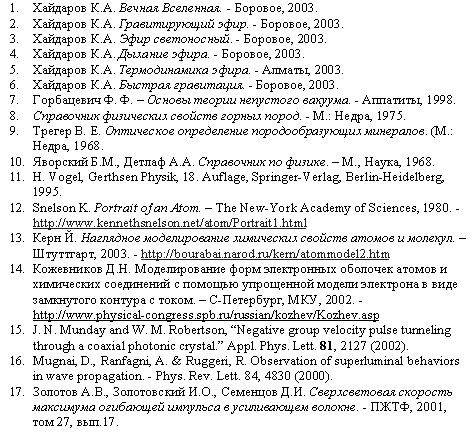Karim Khaidarov
Borovoye, January 30, 2004.
Date of registerred priority: January 30, 2004.

main page   list of papers   library